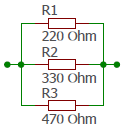# Resistors in parallel

Description of how to calculate resistors in parallel

## Resistors in parallel

If the current in a circuit is distributed over several resistors we call this a parallel circuit of resistors. There is the same voltage across all resistors.If the total current and voltage are known, we can calculate the total resistance according to Ohm's law from the following formula.

$$\displaystyle R_{ges}=\frac{U}{I}$$

If the values of current or voltage are unknown, we can add the conductivities of the individual resistors.

$$\displaystyle G_{ges}=G_1+G_2+G_3$$   das entspricht   $$\displaystyle \frac{1}{R_{ges}}=\frac{1}{R_1}+\frac{1}{R_2}+\frac{1}{R_3}$$

Example with three resistors of $$220$$, $$330$$ and $$470$$ Ohm$$\displaystyle \frac{1}{R_{ges}}=\frac{1}{R_1}+\frac{1}{R_2}+\frac{1}{R_3}= \frac{1}{220}+\frac{1}{330}+\frac{1}{470}=0.0097$$
$$\displaystyle R_{ges}=\frac{1}{0.0097}=103Ω$$

For a circuit in which two resistors are connected in parallel, we can change to a simpler formula by adding the two fractions

$$\displaystyle \frac{1}{R_{ges}} =\frac{1}{R_1}+\frac{1}{R_2}=\frac{R_1+R_2}{R_1·R_2}$$

This results in

$$\displaystyle R_{ges}=\frac{R_1·R_2}{R_1+R_2}$$

Example
$$\displaystyle R_{ges}=\frac{220·330}{220+330}=132$$